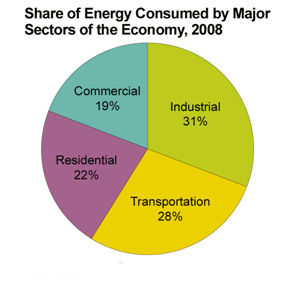FAQ
Home » Blog » FAQ » How to find the KA rating of Circuit breakers?

# How to find the KA rating of Circuit breakers?

Before breaker's selecting for your electrical system, you need to calculate value of expected short circuit current at the place of breaker's installation. Then you need to calculate value of heat pulse and 1s current (expected value of current during one second). After that you need to calculate power of breaker and finally, after all, you can select appropriate breaker. Values of characteristics of selected breaker need to be higher from calculated values of characteristics of your power system.

1. The fault level of the upstream NW (Source) to be known, normally 500MVA or 250MVA.
2. Upstream impedance (reactance and resistor, capacitor to be ignored for Short cct calculation) can be determined accordingly.
3. The LV System starting from the secondary of the distribution transformer.
4. Short circuit percentage voltage for Transformer is known (normally 4% for 1000kVA and 6% for 1500 kVA) and hence reactance and impedance can also determined.
5. Impedance of Cables also can be determined from manufacturer TD sheet.
6. Subtotal impedance to be determined by conventional way (Submit if are in series/ (Z1+Z2+....Zn)/(Z1XZ2X...Zn) if are in parallel.
7. divide voltage by the Impedance up to the required location, will give you the fault current at that location.
8. Determine Maximum and minimum fault current. By the former you can decide the breaking capacity of CB and by the later the setting can be achieved.
9. verify the thermal constraints of the conductors(cables). ie

I²t ≤ S²K² , I short cct current, t time( < 5 s valid), K cable material Factor and S cable section area.
I²t Known as let through energy. accordingly breaking capacity of CB should be > than Circuit Maximum fault.

The MCB, MCCB, & ACB are all Low Voltage Circuit Breakers, where SF6 is a Non-active gaze used in Medium Voltage Circuit Breakers.

Now, to determine the value of Breaking Capacity of any circuit breaker, we should, by calculation, the Maximum Short Circuit Current Value " Isc3max " at the installation point of that circuit breaker, where we can calculate it by assuming a " Short Circuit between 3 phases at that point ", then after knowing " Isc3max " we can determine the Breaking Capacity value that should be " equal or bigger than Isc3max ".

Further:
1- The value " 250 ... 500MVA " is the short circuit power at Medium voltage side for up to 36kV.
2- About the Short Circuit Voltage percentage value:  we called " Ucc or Usc " and the value is " 4& for up to 630kVA transformers ", and " 6% for up to 2500kVA transformers ", but in all case, we can read it at the transformer's name plate.
3- Sorry Mr. Omar, we can't do, you mentioned, the sum of all Z, as these values aren't on the same vector, so, we should first calculate " R & X " for each component, then do the sum of all R " R total " and all X " X total ", then calculate the " Z total ".
4- By knowing the Minimum Short Circuit Current value " Iscmin ", we use it to determine the value of "Setting Value" of "Magnetic Protection or Short-time Protection".

Calculate (4 * 1) =

You may also like:

After calculation of Qc, the choosing of Capacitors type will be done according to the Harmonic Distortion percentage. Noting that in some case where the Harmonic Distortion percentage is high, we should use " ...
I work across the scale of power electronics in voltages and currents. From switchers of 1W for powering ICs to 3kW telco power supplies up to multi-megawatt power converters for reactive power control in AC ...
If you look back over history you will find how things started out from the early engineers and scientists looking at materials and developing systems that would meet their transmission goals. I recall when ...
A 1:1 ratio transformer is primarily used to isolate the primary from the secondary. In small scale electronics it isolates the noise / interference collected from the primary from being transmitted to the ...
Variable frequency drive includes main circuit, power circuit, IPM drive and protection circuits, cooling fan and other several parts. The structure is mostly unitized or modular. Incorrect or unreasonable ...Gozuk Blog: all about electric motor control & drives industries development in energy saving applications.

Featured

Like pumps, fans consume significant electrical energy while serving several applications. In many plants, the VFDs (variable ...A frequency inverter controls AC motor speed. The frequency inverter converts the fixed supply frequency (60 Hz) to a ... Motor starter (also known as soft starter, motor soft starter) is a electronic device integrates soft start, soft stop, ... Soft starter allows the output voltage decreases gradually to achieve soft stop, in order to protect the equipment. Such as the ... Soft Starter reduces electric motor starting current to 2-4 times during motor start up, reduces the impact to power grid during ...

In Discussion